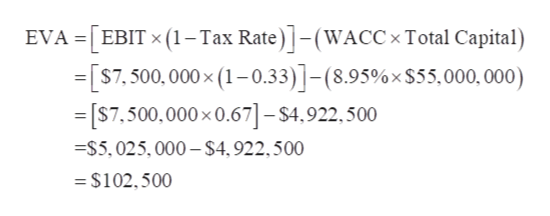# A firm has EBIT of \$7.5 million, a 33% tax rate, a weighted average cost of capital of 8.95% and total capital (long-term debt and equity) of \$55 million. What is their economic value added (EVA)? \$102,500\$112,667\$165,500\$3,264,250

Question
2 views
• A firm has EBIT of \$7.5 million, a 33% tax rate, a weighted average cost of capital of 8.95% and total capital (long-term debt and equity) of \$55 million. What is their economic value added (EVA)?

1. \$102,500
2. \$112,667
3. \$165,500
4. \$3,264,250
check_circle

Step 1

EBIT = \$7.5 Million

Tax Rate = 33%

Weighted Average Cost of Capital (WACC) = 8.95%

Total Capital = \$55 ...help_outlineImage TranscriptioncloseEVA =| EBIT x (1–Tax Rate) |–(WACC × Total Capital) =[S7, 500,000 × (1–0.33)]-(8.95%×\$55,000, 000) = [S7,500,000 x0.67]– \$4.922.500 =S5,025, 000 –- \$4,922,500 = \$102,500 fullscreen

### Want to see the full answer?

See Solution

#### Want to see this answer and more?

Solutions are written by subject experts who are available 24/7. Questions are typically answered within 1 hour.*

See Solution
*Response times may vary by subject and question.
Tagged in